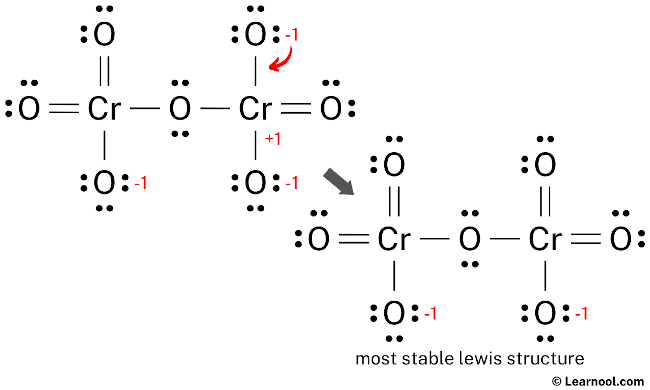# Cr2O72- Lewis structure

Cr2O72- (dichromate) has two chromium atoms and seven oxygen atoms.

In the Cr2O72- Lewis structure, there are two single bonds around the center oxygen atom, with two chromium atoms attached to it, and each chromium makes two single bonds and two double bonds with other three oxygen atoms. The oxygen atom with single bonds has three lone pairs, the oxygen atom with double bonds has two lone pairs, and the center oxygen atom also has two lone pairs.

Also, there is a negative (-1) charge on the two oxygen atoms with single bonds.

Contents

## Steps

To properly draw the Cr2O72- Lewis structure, follow these steps:

#1 Draw a rough sketch of the structure
#2 Next, indicate lone pairs on the atoms
#3 Indicate formal charges on the atoms, if necessary
#4 Minimize formal charges by converting lone pairs of the atoms
#5 Repeat step 4 if necessary, until all charges are minimized

Let’s break down each step in more detail.

### #1 Draw a rough sketch of the structure

• First, determine the total number of valence electrons

In the periodic table, chromium is a transition metal having an electron configuration [Ar] 3d54s1, so chromium has six valence electrons. And oxygen lies in group 16, so oxygen also has six valence electrons.

Since Cr2O72- has two chromium atoms and seven oxygen atoms, so…

Valence electrons of two chromium atoms = 6 × 2 = 12
Valence electrons of seven oxygen atoms = 6 × 7 = 42

Now the Cr2O72- has a negative (-2) charge, so we have to add two more electrons.

So the total valence electrons = 12 + 42 + 2 = 56

• Second, find the total electron pairs

We have a total of 56 valence electrons. And when we divide this value by two, we get the value of total electron pairs.

Total electron pairs = total valence electrons ÷ 2

So the total electron pairs = 56 ÷ 2 = 28

• Third, determine the central atom

We have to place the least electronegative atom at the center.

Since chromium is less electronegative than oxygen, assume that the central atom is chromium.

Here, there are two chromium atoms, so we can assume any one as the central atom.

Let’s assume that the central atom is right chromium.

Therefore, place chromiums in the center and oxygens on either side.

• And finally, draw the rough sketch

### #2 Next, indicate lone pairs on the atoms

Here, we have a total of 28 electron pairs. And eight Cr — O bonds are already marked. So we have to only mark the remaining twenty electron pairs as lone pairs on the sketch.

Also remember that chromium is a period 4 element, so it can keep more than 8 electrons in its last shell. And oxygen is a period 2 element, so it can not keep more than 8 electrons in its last shell.

Always start to mark the lone pairs from outside atoms. Here, the outside atoms are oxygens.

So for center oxygen, there are two lone pairs. For the remaining six oxygens, there are three lone pairs (for each), and for chromium, there is zero lone pair because all twenty electron pairs are over.

Mark the lone pairs on the sketch as follows:

### #3 Indicate formal charges on the atoms, if necessary

Use the following formula to calculate the formal charges on atoms:

Formal charge = valence electrons – nonbonding electrons – ½ bonding electrons

For each chromium atom, formal charge = 6 – 0 – ½ (8) = +2

For center oxygen atom, formal charge = 6 – 4 – ½ (4) = 0

For remaining six oxygen atoms, formal charge = 6 – 6 – ½ (2) = -1

Here, both chromium and oxygen atoms have charges, so mark them on the sketch as follows:

The above structure is not a stable Lewis structure because both chromium and oxygen atoms have charges. Therefore, reduce the charges (as below) by converting lone pairs to bonds.

### #4 Minimize formal charges by converting lone pairs of the atoms

Convert a lone pair of the left oxygen atom to make a new Cr — O bond with the left chromium atom as follows:

### #5 Repeat step 4 (minimize charges again)

Since there are charges on chromium and oxygen atoms, again convert a lone pair of the top oxygen atom to make a new Cr — O bond with the left chromium atom as follows:

### #6 Minimize charges again

There are still charges on chromium and oxygen atoms, so again convert a lone pair of the right oxygen atom to make a new Cr — O bond with the right chromium atom as follows:

### #7 Minimize charges again

There are still charges on chromium and oxygen atoms, so again convert a lone pair of the top oxygen atom to make a new Cr — O bond with the right chromium atom as follows:Lone pair of top right oxygen is converted, and got the most stable Lewis structure of Cr2O72-

In the above structure, you can see that the central atom (right chromium) forms an octet. And the outside atoms (left chromium and oxygens) also form an octet. Hence, the octet rule is satisfied.

Now there is still a negative (-1) charge on the two oxygen atoms.

This is okay, because the structure with a negative charge on the most electronegative atom is the best Lewis structure. And in this case, the most electronegative element is oxygen.

Also, the above structure is more stable than the previous structures. Therefore, this structure is the most stable Lewis structure of Cr2O72-.

And since the Cr2O72- has a negative (-2) charge, mention that charge on the Lewis structure by drawing brackets as follows: